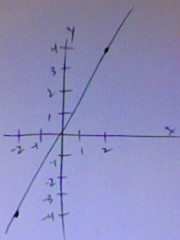Show Me How To Graph Y=2x?

There are two ways to draw graphs, either manually (with pen and paper) or electronically. I will explain how to use both methods.

If you are drawing it manually you will need a sheet of squared paper (either normal mathematics paper, or graph paper). Then label the two axis (x, horizontal and y, vertical) between a suitable range of values, my suggest would be ± 5 for the x axis and ± 10 for the y axis. After this, draw a table with x and y as the headings. Fill in the values for x and y at each integer values. These will be (-5,-10), (-4,-8) ... (+4,+8), (+5,+10). You can then plot these on your graph paper and draw a line through the points. This will be the line y = 2x.
Alternatively, you can use a graphing program. There are two types of program, internet based applets, and installed computer programs. A website like wolframalpha.com is an example of a applet that you can use to graph things for you, along with a lot more. If you enter "plot y=2x" it will graph it for you.
If you experienced with graphing programs, you can use a computer program such as autograph (£59, autograph-math.com, windows and Mac). Click "new 2D sheet", right click and select "new equation" then enter "y=2x" and press enter. You can then see the graph. If you have a computer with OS x loaded already you will find a free graphing program called "grapher" in the utilities folder. Choose default 2D graph and enter y=2x into the bar at the top and press enter.

Once you have graphed these you can see any point on the graph, and play around with it: Zoom in and out, draw extra lines on top of it, etc...
thanked the writer.